# GMAT Math : Counting Methods

## Example Questions

← Previous 1

### Example Question #131 : Arithmetic

Oteri can choose to wear jeans, sweat pants, or shorts, a button down or a tee shirt, and a hat or no hat. How many different outfit combinations does he have in his wardrobe?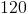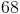Explanation:

He has 3 pants, 2 shirts, 2 hat choices (hat or no hat).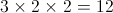### Example Question #1 : Dsq: Understanding Counting Methods

Olivia picksballs, each one after the other, from a basket containingballs.

How many possible combinations of balls can she end up with?

(1)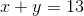(2)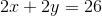Statement (1) ALONE is sufficient, but statement (2) alone is not sufficient

Statement (2) ALONE is sufficient, but statement (1) alone is not sufficient

Statements (1) and (2) TOGETHER are not sufficient

EACH statement ALONE is sufficient

BOTH statements TOGETHER are sufficient, but NEITHER statement ALONE is sufficient

Statements (1) and (2) TOGETHER are not sufficient

Explanation:

Each statement on its own gives us one equation with 2 unknowns, which is unsolvable. Therefore each statement alone is not sufficient.

The two equations are equivalent: x + y = 13 and 2.(x+y) = 2 x 13 = 26

Therefore we cannot sole for x and y either with both equations.

### Example Question #3 : Dsq: Understanding Counting Methods

A Lunch Deal Meal at the Chinese restaurant where Jack likes to eat comprises one appetizer, one entree, and one beverage. How many possible Lunch Meal Deals can Jack choose from?

Statement 1: Jack can choose from seven appetizers and ten entrees.

Statement 2: Jack can choose from as many beverages as appetizers.

BOTH statements TOGETHER are sufficient to answer the question, but NEITHER statement ALONE is sufficient to answer the question.

BOTH statements TOGETHER are insufficient to answer the question.

Statement 2 ALONE is sufficient to answer the question, but Statement 1 ALONE is NOT sufficient to answer the question.

Statement 1 ALONE is sufficient to answer the question, but Statement 2 ALONE is NOT sufficient to answer the question.

EITHER statement ALONE is sufficient to answer the question.

BOTH statements TOGETHER are sufficient to answer the question, but NEITHER statement ALONE is sufficient to answer the question.

Explanation:

By the multiplication principle, the number of meals Jack can make is the product of the number of appetizers, the number of entrees, and the number of beverages. Statement 1 tells only the first two; Statement 2 does not give any of these quantities. From the two statements together, we know that there are seven appetizers, ten entrees, and seven beverages, so there are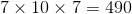possible Lunch Deal Meals.

### Example Question #1 : Dsq: Understanding Counting Methods

A Veggie Platter at the restaurant where Lucy likes to eat comprises four different vegetables. How many possible Veggie Platters can Lucy choose from?

Statement 1: The Veggie Platter costs $5.29 plus tax. Statement 2: The menu from which Lucy may choose her vegetables is below: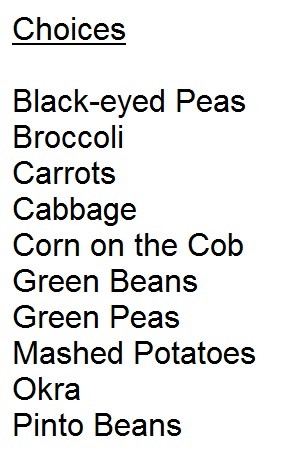Possible Answers: BOTH statements TOGETHER are sufficient to answer the question, but NEITHER statement ALONE is sufficient to answer the question. BOTH statements TOGETHER are insufficient to answer the question. Statement 1 ALONE is sufficient to answer the question, but Statement 2 ALONE is NOT sufficient to answer the question. Statement 2 ALONE is sufficient to answer the question, but Statement 1 ALONE is NOT sufficient to answer the question. EITHER statement ALONE is sufficient to answer the question. Correct answer: Statement 2 ALONE is sufficient to answer the question, but Statement 1 ALONE is NOT sufficient to answer the question. Explanation: Statement 1 alone gives irrelevant information; the cost of the Veggie Platter has no bearing on the number of possible combinations. From Statement 2 alone, it can be determined that there are ten choices; therefore, the number of ways Lucy can order a Veggie Platter can be determined to be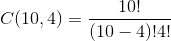. ### Example Question #1 : Dsq: Understanding Counting Methods A Veggie Platter at the restaurant where Georgia likes to eat comprises four different vegetables. How many possible Veggie Platters can Georgia choose from? Statement 1: Half the choices are listed as being "hearth healthy". Statement 2: Seven of the choices are not listed as being "hearth healthy". Possible Answers: Statement 1 ALONE is sufficient to answer the question, but Statement 2 ALONE is NOT sufficient to answer the question. BOTH statements TOGETHER are sufficient to answer the question, but NEITHER statement ALONE is sufficient to answer the question. EITHER statement ALONE is sufficient to answer the question. BOTH statements TOGETHER are insufficient to answer the question. Statement 2 ALONE is sufficient to answer the question, but Statement 1 ALONE is NOT sufficient to answer the question. Correct answer: BOTH statements TOGETHER are sufficient to answer the question, but NEITHER statement ALONE is sufficient to answer the question. Explanation: The only thing needed in order to answer the question is the number of vegetables from which Georgia has to choose. Neither statement alone gives this information. However, it is possible to deduce this information from both statements together. From Statement 1, half the choices are "hearth healthy"; therefore, half are not. From Statement 2, this amounts to seven choices, so the total number of vegetables on the menu is fourteen, and there are a total of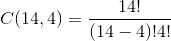possible Veggie Platters. ### Example Question #6 : Dsq: Understanding Counting Methods A Veggie Platter at the restaurant where George likes to eat comprises four different vegetables. How many possible Veggie Platters can George choose from? Statement 1: George can choose from ten vegetables. Statement 2: The choices are black-eyed peas, corn on the cob, and mashed potatoes, among seven others. Possible Answers: BOTH statements TOGETHER are sufficient to answer the question, but NEITHER statement ALONE is sufficient to answer the question. EITHER statement ALONE is sufficient to answer the question. BOTH statements TOGETHER are insufficient to answer the question. Statement 2 ALONE is sufficient to answer the question, but Statement 1 ALONE is NOT sufficient to answer the question. Statement 1 ALONE is sufficient to answer the question, but Statement 2 ALONE is NOT sufficient to answer the question. Correct answer: EITHER statement ALONE is sufficient to answer the question. Explanation: Each statement alone says that George can choose from ten vegetables - Statement 2 is different only in that it names three of them. George can choose any one of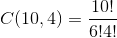different Veggie Platters. ### Example Question #7 : Dsq: Understanding Counting Methods A Lunch Deal Meal at the Chinese restaurant where Mickey likes to eat comprises one appetizer, one entree, and one beverage. How many possible Lunch Meal Deals can Mickey choose from? Statement 1: The number of appetizers and the number of beverages from which Mickey may choose are both equal to the number of entrees that do not have shrimp. Statement 2: Mickey may choose any of ten entrees, but if he chooses any of the three entrees with shrimp, he must pay an upcharge of$1.

BOTH statements TOGETHER are sufficient to answer the question, but NEITHER statement ALONE is sufficient to answer the question.

Statement 2 ALONE is sufficient to answer the question, but Statement 1 ALONE is NOT sufficient to answer the question.

Statement 1 ALONE is sufficient to answer the question, but Statement 2 ALONE is NOT sufficient to answer the question.

EITHER statement ALONE is sufficient to answer the question.

BOTH statements TOGETHER are insufficient to answer the question.

BOTH statements TOGETHER are sufficient to answer the question, but NEITHER statement ALONE is sufficient to answer the question.

Explanation:

By the multiplication principle, the number of possible Lunch Deal Meals is the product of the number of appetizers, the number of entrees, and the number of beverages. Neither statement alone gives all three numbers.

Assume both statements are true. From Statement 2, there are ten entrees on the menu, seven of which do not have shrimp (note that the upcharge is irrelevant). From Statement 1, the number of appetizers and the number of beverages are both equal to the number of entrees that do not have shrimp, which is seven. Therefore, the number of possible Lunch Deal Meals is.

### Example Question #1 : Dsq: Understanding Counting Methods

A Veggie Platter at the restaurant where Steve likes to eat comprises four different vegetables. How many possible Veggie Platters can Steve choose from?

Statement 1: The menu from which Steve may choose his vegetables is below:Statement 2: If Steve orders corn on the cob or broccoli, he will pay an upcharge; if he chooses only from the remaining eight vegetables, he will pay the regular price.

BOTH statements TOGETHER are insufficient to answer the question.

Statement 2 ALONE is sufficient to answer the question, but Statement 1 ALONE is NOT sufficient to answer the question.

Statement 1 ALONE is sufficient to answer the question, but Statement 2 ALONE is NOT sufficient to answer the question.

EITHER statement ALONE is sufficient to answer the question.

BOTH statements TOGETHER are sufficient to answer the question, but NEITHER statement ALONE is sufficient to answer the question.

EITHER statement ALONE is sufficient to answer the question.

Explanation:

From either statement alone, it can be determined that there are ten choices; Statement 1 lists them, and Statement 2, while giving some irrelevant information, still gives this number, albeit a bit obliquely ("corn on the cob or broccoli", "the other eight choices"). Therefore, the number of ways Steve can order a Veggie Platter can be determined to be.

### Example Question #9 : Dsq: Understanding Counting Methods

A Lunch Deal Meal at the restaurant where Allen likes to eat comprises one appetizer, one entree, and one beverage. How many possible Lunch Meal Deals can Allen choose from?

Statement 1: Allen can choose iced tea, cola, milk, or any of nine other beverages.

Statement 2: Allen can choose General Tso's chicken, Mu Shu pork, egg foo young, or any of seven other entrees.

BOTH statements TOGETHER are insufficient to answer the question.

EITHER statement ALONE is sufficient to answer the question.

BOTH statements TOGETHER are sufficient to answer the question, but NEITHER statement ALONE is sufficient to answer the question.

Statement 2 ALONE is sufficient to answer the question, but Statement 1 ALONE is NOT sufficient to answer the question.

Statement 1 ALONE is sufficient to answer the question, but Statement 2 ALONE is NOT sufficient to answer the question.

BOTH statements TOGETHER are insufficient to answer the question.

Explanation:

By the multiplication principle, the number of possible Lunch Deal Meals is the product of the number of appetizers, the number of entrees, and the number of beverages. The two statements together give us the number of entrees and the number of beverages, but not the number of appetizers.

### Example Question #2 : Dsq: Understanding Counting Methods

A Veggie Platter at the restaurant where Maggie likes to eat comprises four different vegetables. How many possible Veggie Platters can Maggie choose from?

Statement 1: The Veggie Platter costs $6.19 plus tax if Maggie selects a baked potato as one of her choices, and$5.79 plus tax if Maggie chooses only from the remaining eleven choices.

Statement 2: Maggie has ordered corn on the cob every time she has ordered a Veggie Platter.

Statement 1 ALONE is sufficient to answer the question, but Statement 2 ALONE is NOT sufficient to answer the question.

BOTH statements TOGETHER are sufficient to answer the question, but NEITHER statement ALONE is sufficient to answer the question.

Statement 2 ALONE is sufficient to answer the question, but Statement 1 ALONE is NOT sufficient to answer the question.

EITHER statement ALONE is sufficient to answer the question.

BOTH statements TOGETHER are insufficient to answer the question.

Statement 1 ALONE is sufficient to answer the question, but Statement 2 ALONE is NOT sufficient to answer the question.

Explanation:

Statement 1 gives the number of vegetables from which Maggie may choose, albeit somewhat obliquely ("baked potato", "the remaining eleven choices") and along with other, irrelevant information. Therefore, the number of ways Maggie can order a Veggie Platter can be determined to be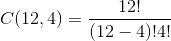.

Statement 2 alone is irrelevant, since the question asks for the total number of possible meals. What Maggie has actually ordered in the past has no bearing on the answer.

← Previous 1

### All GMAT Math Resources